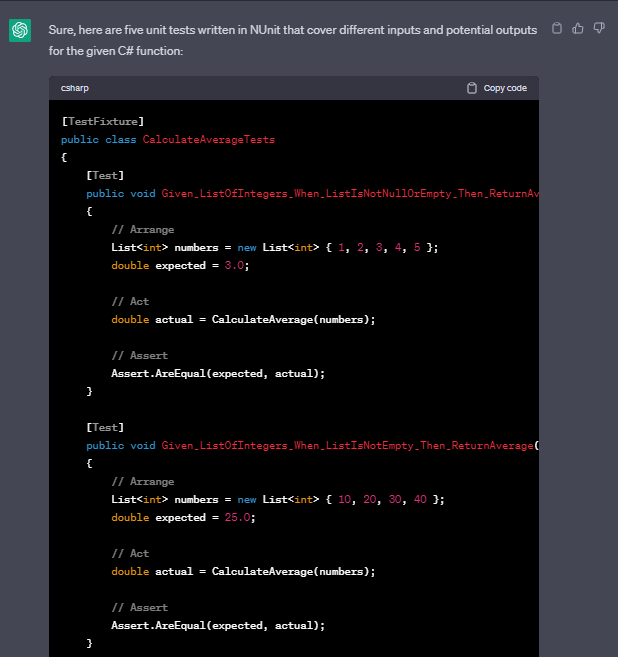# Unit Testing with ChatGPTAutomated testing is an essential part of software development, enabling faster feedback and higher quality. Unit testing is an subset of automated testing, aimed at testing the smallest testable units of software, often individual functions or methods.

However, writing unit tests can be a challenge and sometimes time-consuming, especially when dealing with edge cases and a lot of different inputs and outputs.

## Hello ChatGPT! 🤖

ChatGPT can be trained to generate these test cases and assertions that cover a lot of different cases. These generated tests can then be used as a starting point for developers to refine and improve the quality of their own unit tests.

In this blog post, I will explain how ChatGPT can be utilized to generate unit tests for C# code, saving developers time and improving testing efficiency.

Note: In the unlikely event that you don't know what ChatGPT is then I suggest you read up on this before reading any further.

## Lets get started!

To demonstrate the use of ChatGPT in generating unit tests, let's consider a simple example of testing a function that calculates the average of a list of integers:

```public double CalculateAverage(List numbers)
{
if (numbers == null || numbers.Count == 0)
{
throw new ArgumentException("List of numbers cannot be empty or null");
}
int sum = 0;
foreach (int num in numbers)
{
sum += num;
}
return sum / numbers.Count;
}
```

Sidenote: I didn't even write this code myself, I asked ChatGPT for a good piece of code for this blogpost purpose. 😎

## Step 1: List inputs and outputs.

First we feed ChatGPT with this function along with some instructions to give us five potential input and outputs, which might look something like this:

Request:

```Based on this C# function.
List 5 different outputs based on various inputs.
Make sure to include null numbers as a potential input.```

Response:

```Sure, here are five different outputs based on various inputs for the given C# function, including null numbers as a potential input:

Input: { 1, 2, 3, 4, 5 }
Output: 3.0

Input: { 10, 20, 30, 40 }
Output: 25.0

Input: { -10, 0, 10 }
Output: 0.0

Input: { 0, 0, 0, 0, 0 }
Output: 0.0

Input: null
Output: System.ArgumentException: List of numbers cannot be empty or null.```

## Step 2: Generating the tests

Next we tell ChatGPT to generate five unit tests that will cover these inputs and outputs, along with some instructions on which test framework we want to use and preferred naming convention:

Request:

```Based on this C# function.
Give me five Unit Tests that covers these inputs and potential outputs.
The tests should be using NUnit and follow a naming standard of Given_x_When_y_Then_z.```

And this is what ChatGPT returned. It's worth noting that this simple C# function where relatively straightforward. In more complex code, you may need to tweak the messages you provide to ChatGPT with more context or specific test cases based on your acceptance criteria.

Response:

```Sure, here are five unit tests written in NUnit that cover different inputs and potential outputs for the given C# function:

public class CalculateAverageTests
{
[Test]
public void Given_ListOfIntegers_When_ListIsNotNullOrEmpty_Then_ReturnAverage()
{
// Arrange
List numbers = new List { 1, 2, 3, 4, 5 };
double expected = 3.0;

// Act
double actual = CalculateAverage(numbers);

// Assert
Assert.AreEqual(expected, actual);
}

[Test]
public void Given_ListOfIntegers_When_ListIsNotEmpty_Then_ReturnAverage()
{
// Arrange
List numbers = new List { 10, 20, 30, 40 };
double expected = 25.0;

// Act
double actual = CalculateAverage(numbers);

// Assert
Assert.AreEqual(expected, actual);
}

[Test]
public void Given_ListOfIntegers_When_ListHasNegativeNumbers_Then_ReturnAverage()
{
// Arrange
List numbers = new List { -10, 0, 10 };
double expected = 0.0;

// Act
double actual = CalculateAverage(numbers);

// Assert
Assert.AreEqual(expected, actual);
}

[Test]
public void Given_ListOfIntegers_When_ListHasAllZeroes_Then_ReturnZero()
{
// Arrange
List numbers = new List { 0, 0, 0, 0, 0 };
double expected = 0.0;

// Act
double actual = CalculateAverage(numbers);

// Assert
Assert.AreEqual(expected, actual);
}

[Test]
public void Given_NullList_When_NullListIsPassed_Then_ThrowArgumentException()
{
// Arrange
List numbers = null;

// Act & Assert
Assert.Throws(() => CalculateAverage(numbers),
"List of numbers cannot be empty or null");
}
}```

In this example, ChatGPT has generated five test cases that cover different input scenarios and edge cases based on a provided C# function. The generated tests provide a good starting point for developers to refine and improve the quality of their own unit tests.

It's worth noting that generating unit tests using ChatGPT is not a substitute for manual testing and code review. Developers still need to carefully examine the generated tests to ensure their correctness and coverage.

"While ChatGPT-generated tests may not be perfect, they can be an excellent starting point for developers looking to improve their code coverage and overall testing efficiency."

## Conclusion:

In conclusion, ChatGPT is a promising tool for generating unit tests for C# code, providing developers with a time-saving and efficient approach to test code functionality. While ChatGPT-generated tests may not be perfect, they can be an excellent starting point for developers looking to improve their code coverage and overall testing efficiency.

Cheers friends! ❤️